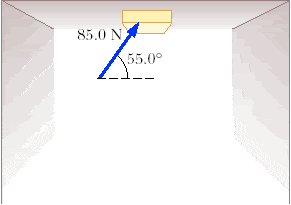# Determining coefficient of kinetic friction help!

A 3.40 kg block is pushed along the ceiling with a constant applied force of 85.0 N that acts at an angle of 55.0° with the horizontal, as in the figure below. The block accelerates to the right at 6.00 m/s2. Determine the coefficient of kinetic friction between block and ceiling.Ugghhh so... this is what I did...

F = 85.0 N
m = 3.40 kg
a = 6.00 m/s2
fk = μkn

1) Forces in the x-direction:
F cos θ
fk (kinetic friction)

Forces in the y-direction:
F sin θ
n (normal force)

2) Sum of forces

ƩFx = F cos θ + fk = 0
ƩFy = F sin θ + n = 0
ƩFy = F sin θ + n = 0
ƩFy = n = -F sin θ

3) ƩFx = F cos θ + μkn = 0
3) ƩFx = F cos θ + μk(-F sin θ) = 0
3) ƩFx = F cos θ + μk(-F sin θ) = 0
3) ƩFx = 85 cos 55 - μk(85 sin 55) = 0
3) ƩFx = 85 cos 55 = μk(85 sin 55)
3) ƩFx = (85 cos 55)/(85 sin 55) = μk
3) ƩFx = 0.700 = μk

So I got this wrong, the answer is actually 0.781 (???)

I don't know what to do!

A 3.40 kg block is pushed along the ceiling with a constant applied force of 85.0 N that acts at an angle of 55.0° with the horizontal, as in the figure below. The block accelerates to the right at 6.00 m/s2. Determine the coefficient of kinetic friction between block and ceiling
-----------------------
It is accelerating, net Fx not zero.
The force applied is pressing the block to the ceiling. The weight is pulling it away.
I got this value - 0.78093138

Last edited:
A 3.40 kg block is pushed along the ceiling with a constant applied force of 85.0 N that acts at an angle of 55.0° with the horizontal, as in the figure below. The block accelerates to the right at 6.00 m/s2. Determine the coefficient of kinetic friction between block and ceiling
-----------------------
It is accelerating, net Fx not zero.
The force applied is pressing the block to the ceiling. The weight is pulling it away.
I got this value - 0.78093138

So is it supposed to be this???

ƩFx = F cos θ + μkn = 6

85 cos 55 + μk(-85 sin 55) = 6
85 cos 55 - μk(85 sin 55) = 6
85 cos 55 = 6 + μk(85 sin 55)
48.75 - 6 = 69.62μk
42.75/69.62 = μk
0.614 = μk

-____-

Can you please show me what you did?

Last edited:
Force of kinetic friction:
fk = μkn

Horizontal force of acceleration in the x-direction/Value of the bottom leg of the triangle:
Facceleration-x = Fa(cos θ)
Facceleration-x = 85(cos 55)
Facceleration-x = 48.75 N

Normal force n (value of the upright, vertical leg of the triangle):
n = Facceleration-y(sin θ) + mg
n = 85(sin 55) + (3.40 kg)(6.00 m/s2)
n = 69.62 + 20.4
n = 90.02 N

fk = μkn
48.75 = μk(90.02)
48.75/90.02 = μk
0.541 = μk

Help?

A 3.40 kg block is pushed along the ceiling with a constant applied force of 85.0 N that acts at an angle of 55.0° with the horizontal, as in the figure below. The block accelerates to the right at 6.00 m/s2. Determine the coefficient of kinetic friction between block and ceiling
-----------------------
It is accelerating, net Fx not zero.
The force applied is pressing the block to the ceiling. The weight is pulling it away.
I got this value - 0.78093138

You were completely wrong.
Net force doesn't equal 6.
Thanks for nothing.

You were completely wrong.
Net force doesn't equal 6.
Thanks for nothing.

I not sure whether you read the question.
I just copy and paste the original question you posted and just underlined it.

A 3.40 kg block is pushed along the ceiling with a constant applied force of 85.0 N that acts at an angle of 55.0° with the horizontal, as in the figure below. The block accelerates to the right at 6.00 m/s2. Determine the coefficient of kinetic friction between block and ceiling.

Here your original question and i underlined it.